Thursday , July 7 2022# NCERT 5th Class (CBSE) Mathematics: Area And Volume

Put in as many layers as required to fill the box up to the top.

There are 10 layers of 96 cubes.

There are 960 cubes in the box.

Since the box can hold 960, 1 cm cubes, the volume of the box is 960 cubic centimeters.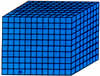The volume of an object is measured by the number of cubic units needed to fill in the object.

The unit of measurement of volume can be cubic millimeters, cubic centimeters or cubic meters.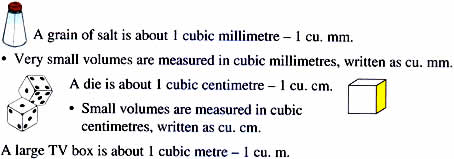• Large volumes are measured in cubic meters, written as cu. m.

(a) Find the volume of this cube if each small cube is 1 cu. cm.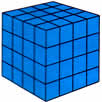One layer = 4 × 4 cubes = 16 cubes

4 layers = 16 × 4 = 64 cu. cm

(b) Find the volume of this solid. Each cube is 1 cu. cm.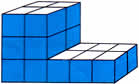Top layer = 4 cubes

Middle layer = 4 cubes

Bottom layer = 10 cubes

Cubes in all = 18 cubes

Volume = 18 cu. cm.

### Measuring by multiplying

Now let us measure the volume of this box using a shortcut.

Step 1: Find the cubes per layer by multiplying the number of cm cubes used in the length by the number of cm cubes used in the breadth.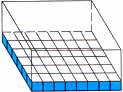Length = 8 cubes